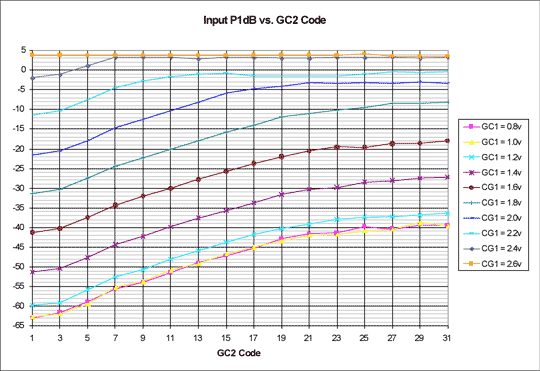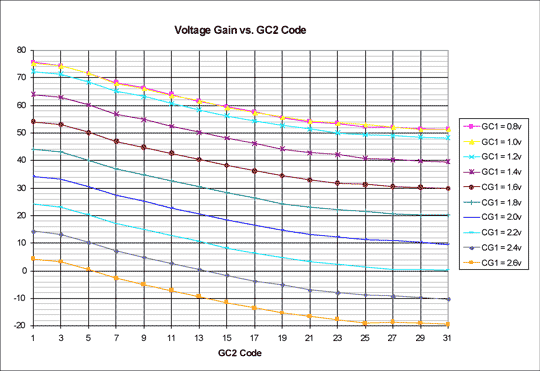# P1dB Characterization for the MAX2116 Satellite Tuner

### Abstract

P1dB measurement data is provided for the MAX2116 direct conversion tuner at particular combinations of RF and baseband gain settings throughout their ranges. Voltage gain plots quantify gain distribution corresponding to these particular gain settings. Together, the P1dB and voltage gain plots facilitate system design tradeoffs.

### Introduction

P1dB measurement data is provided for the MAX2116 at particular combinations of RF and baseband gain settings throughout their ranges. This data is valuable for selecting gain-control settings that properly distribute front-end and back-end gain for adequate P1dB performance. In conjunction with noise-figure data, these measurements are useful for optimizing the dynamic range of the receiver system.

For large received signals, high P1dB is required to avoid saturation of the tuner. By adjusting the RF variable-gain amplifier for lower gain, the P1dB is increased significantly. This enables reception of large signals. Since this reduced RF gain increases the tuner noise figure, careful system design tradeoffs are required between P1dB and noise figure. This application note is a tool to calculate these tradeoffs.

### Operational Overview

Figure 1 shows a typical operating circuit for the MAX2116. Pins 4 and 5 are the differential RF input. At large-input signal levels, the output amplifiers will limit the Carrier to Noise Ratio (C/N). One of the limiting factors is P1dB. At low-input signal levels, system noise figure will limit the tuner's C/N.

Pin 7 (GC1) is the variable gain control for the RF front-end. The gain control line is typically controlled by a filtered PWM signal. A baseband demodulator IC generates the PWM signal. In a closed-loop system the filtered PWM output will force a constant amplitude signal on the MAX2116 IQ output.

Pins 37 and 34 on the MAX2116 are the I/Q outputs. The single-ended output amplifier gains can be set to deliver 800mVP-P at the output.

After the mixer, there is a variable-gain baseband amplifier. This amplifier's gain is set by register GC2, which is adjustable from 0 to 31 (decimal). System P1dB is set by the particular combination of GC1 and GC2.Figure 1. Typical operating circuit for the MAX2116 direct-conversion tuner.

### Input P1dB MeasurementsFigure 2. Input P1dB vs. GC2 code.

Figure 2 shows the P1dB of the MAX2116 for different RF and baseband gain control settings. The trends of increasing P1dB as the front-end and back-end gains decrease are evident, with the front-end impact dominating. The P1dB limit of about 3.5dBm is reached for any GC2 setting at a minimum front-end gain setting of 2.6V. The data in Figure 2 is measured with a midband carrier-wave RF input at 1550MHz and a 5MHz IF frequency.Figure 3. Voltage gain vs. GC2 code.

Figure 3 shows the MAX2116 voltage gain at different gain-control settings that correspond to those shown in Figure 2 above. The maximum gain is 76dB; the GC1 range is 72dB and the GC2 range is 22dB. Each of these values is near the typical data sheet values.

For further data analysis, you download access an Excel spreadsheet containing the raw data shown in Figures 2 and 3.

### Conclusion

P1dB data is presented throughout the ranges of the MAX2116's RF and baseband gain-control settings. This data is useful for identifying the possible RF and baseband gain distributions that provide adequate P1dB performance.